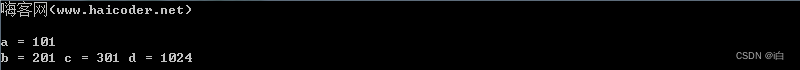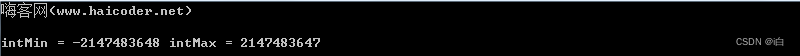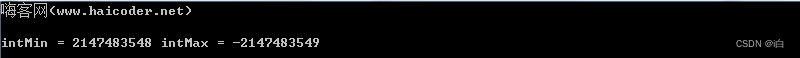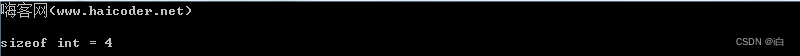# C++ int

## C++ int教程

C++ 中的 int 用来表示一个 整数，也可以叫做整型，int 的取值范围是介于 shortlong 之间的。

## C++ int定义详解

### 语法

``````int varname = value;
``````

### 参数

int 定义 int 类型变量使用的类型。
varname 变量名。
value 可选，变量的初始值，该值不可以超过 int 类型的最大值。

## C++ int详解

### 说明

C++ 的 int 的取值范围为 -2147483648 ~ 2147483647，同时，在 limits.h 中有 常量 INT_MIN 表示其最小值和 INT_MAX 表示其最大值。

## 案例

### 定义int变量

``````#include <iostream>
#include <climits>
using namespace std;

int main(int argc, char **argv)
{
cout << "嗨客网(www.haicoder.net)\n" << endl;

int a = 101;
int b = 201, c = 301, d = 1024;

cout << "a = " << a << endl;
cout << "b = " << b << " c = " << c << " d = " << d << endl;
}
``````### int变量取值范围

``````#include <iostream>
#include <climits>
using namespace std;

int main(int argc, char **argv)
{
cout << "嗨客网(www.haicoder.net)\n" << endl;

int intMin = INT_MIN;
int intMax = INT_MAX;

cout << "intMin = " << intMin << " intMax = " << intMax << endl;
}
``````### int变量超出范围

``````#include <iostream>
#include <climits>
using namespace std;

int main(int argc, char **argv)
{
cout << "嗨客网(www.haicoder.net)\n" << endl;

int intMin = INT_MIN - 100;
int intMax = INT_MAX + 100;

cout << "intMin = " << intMin << " intMax = " << intMax << endl;
}
``````### int字节数

``````#include <iostream>
#include <climits>
using namespace std;

int main(int argc, char **argv)
{
cout << "嗨客网(www.haicoder.net)\n" << endl;

int num = 100;

cout << "sizeof int = " << sizeof(int) << endl;
}
``````## C++ int总结

C++ 的 int 的取值范围为 -2147483648 ~ 2147483647，int 占 4 个字节。

THE END